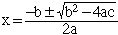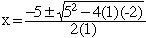I am a student in the UK and i am in secondary school and i am doing my GCSE next year. here are my questions: solve the following equations correct to 2 decimal each time.  2xsquared + 5x = 1  3xsquared(2x+1)=1  xsquared + 7x + 2 = 2x + 4  5xsquared - 3x = xsquared + 4 PS: if it is possible can you answer them by monday night please. Hi Philip, What you need to do in each case is to rearrance the expression so that it is in the form a x2 + b x + c = 0 Thus for example in your problem  x2 + 7x + 2 = 2x + 4 becomes x2 + 7x + 2 - 2x - 4 = 0 or x2 + 5x - 2 = 0 If the exprression a x2 + b x + c that you get can be factored then factor it and solve for x. If not then you can use the general quadratic.Thus for problem  again where a = 1, b = 5 and c = -2,Finally use your calculator to evaluate this exprression. Cheers, Penny Go to Math Central